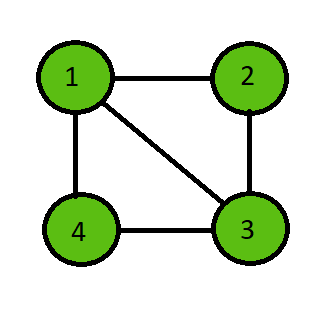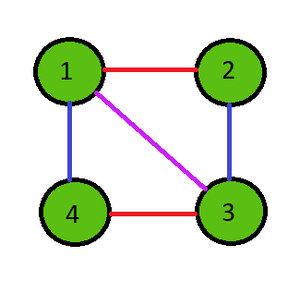# Java Program to Implement the Vizing’s Theorem

Vizing’s Theorem of graph theory states that every simple undirected graph has a chromatic index of one larger than the maximum degree ‘d’ of the graph. In simple meaning, the theorem states that the chromatic index can be either ‘d’ or ‘d’+1.

Chromatic Index of a graph is the minimum number of colors required to color the edges of the graph such that any two edges that share the same vertex have different colors.

Examples:

Input:Output:

Chromatic Index = 3

Edge from 1 to 2 : Color 1

Edge from 2 to 3 : Color 2

Edge from 3 to 4 : Color 1

Edge from 4 to 1 : Color 2

Edge from 1 to 3 : Color 3Algorithm:

Below is the step-by-step approach of the algorithm:-

• Initialize the number of edges and the edge list.
• Color the graph according to the Vizing’s Theorem.
• Assign a color to an edge and check if any adjacent edges have the same color or not.
• If any adjacent edge has the same color, then increment the color to try the next color for that edge.
• Repeat till all the edges get it’s color according to the theorem.
• Once done print the maximum value of color for all the edges and the colors of every edge.

Implementation of the above approach:

## Java

 `// Java program to Implement ` `// Vizing's Theorem ` ` `  `import` `java.util.*; ` ` `  `public` `class` `chromaticIndex { ` ` `  `    ``// Function to find the chromatic index ` `    ``public` `void` `edgeColoring(``int``[][] edges, ``int` `e) ` `    ``{ ` `        ``// Initialize edge to first ` `        ``// edge and color to color 1 ` `        ``int` `i = ``0``, color = ``1``; ` ` `  `        ``// Repeat until all edges are done coloring ` `        ``while` `(i < e) { ` ` `  `            ``// Give the selected edge a color ` `            ``edges[i][``2``] = color; ` ` `  `            ``boolean` `flag = ``false``; ` ` `  `            ``// Iterate through all others edges to check ` `            ``for` `(``int` `j = ``0``; j < e; j++) { ` ` `  `                ``// Ignore if same edge ` `                ``if` `(j == i) ` `                    ``continue``; ` ` `  `                ``// Check if one vertex is similar ` `                ``if` `((edges[i][``0``] == edges[j][``0``]) ` `                    ``|| (edges[i][``1``] == edges[j][``0``]) ` `                    ``|| (edges[i][``0``] == edges[j][``1``]) ` `                    ``|| (edges[i][``1``] == edges[j][``1``])) { ` ` `  `                    ``// Check if color is similar ` `                    ``if` `(edges[i][``2``] == edges[j][``2``]) { ` ` `  `                        ``// Increment the color by 1 ` `                        ``color++; ` `                        ``flag = ``true``; ` `                        ``break``; ` `                    ``} ` `                ``} ` `            ``} ` ` `  `            ``// If same color faced then repeat again ` `            ``if` `(flag == ``true``) { ` `                ``continue``; ` `            ``} ` ` `  `            ``// Or else proceed to a new vertex with color 1 ` `            ``color = ``1``; ` `            ``i++; ` `        ``} ` ` `  `        ``// Check the maximum color from all the edge colors ` `        ``int` `maxColor = -``1``; ` `        ``for` `(i = ``0``; i < e; i++) { ` `            ``maxColor = Math.max(maxColor, edges[i][``2``]); ` `        ``} ` ` `  `        ``// Print the chromatic index ` `        ``System.out.println(``"Chromatic Index = "` `+ maxColor); ` `       `  `        ``for` `(i = ``0``; i < e; i++) ` `        ``{ ` `            ``System.out.println(``"Edge from "` `+ edges[i][``0``] ` `                               ``+ ``" to "` `+ edges[i][``1``] ` `                               ``+ ``" : Color "` `+ edges[i][``2``]); ` `        ``} ` `    ``} ` ` `  `    ``// Driver code ` `    ``public` `static` `void` `main(String[] args) ` `    ``{ ` ` `  `        ``// Number of edges ` `        ``int` `e = ``5``; ` ` `  `        ``// Edge list ` `        ``int``[][] edges = ``new` `int``[e][``3``]; ` ` `  `        ``// Initialize all edge colors to 0 ` `        ``for` `(``int` `i = ``0``; i < e; i++) { ` `            ``edges[i][``2``] = -``1``; ` `        ``} ` ` `  `        ``// Edges ` `        ``edges[``0``][``0``] = ``1``; ` `        ``edges[``0``][``1``] = ``2``; ` ` `  `        ``edges[``1``][``0``] = ``2``; ` `        ``edges[``1``][``1``] = ``3``; ` ` `  `        ``edges[``2``][``0``] = ``3``; ` `        ``edges[``2``][``1``] = ``4``; ` ` `  `        ``edges[``3``][``0``] = ``4``; ` `        ``edges[``3``][``1``] = ``1``; ` ` `  `        ``edges[``4``][``0``] = ``1``; ` `        ``edges[``4``][``1``] = ``3``; ` ` `  `        ``// Run the function ` `        ``chromaticIndex c = ``new` `chromaticIndex(); ` `        ``c.edgeColoring(edges, e); ` `    ``} ` `}`

Output

```Chromatic Index = 3
Edge from 1 to 2 : Color 1
Edge from 2 to 3 : Color 2
Edge from 3 to 4 : Color 1
Edge from 4 to 1 : Color 2
Edge from 1 to 3 : Color 3
```

Whether you're preparing for your first job interview or aiming to upskill in this ever-evolving tech landscape, GeeksforGeeks Courses are your key to success. We provide top-quality content at affordable prices, all geared towards accelerating your growth in a time-bound manner. Join the millions we've already empowered, and we're here to do the same for you. Don't miss out - check it out now!

Previous
Next